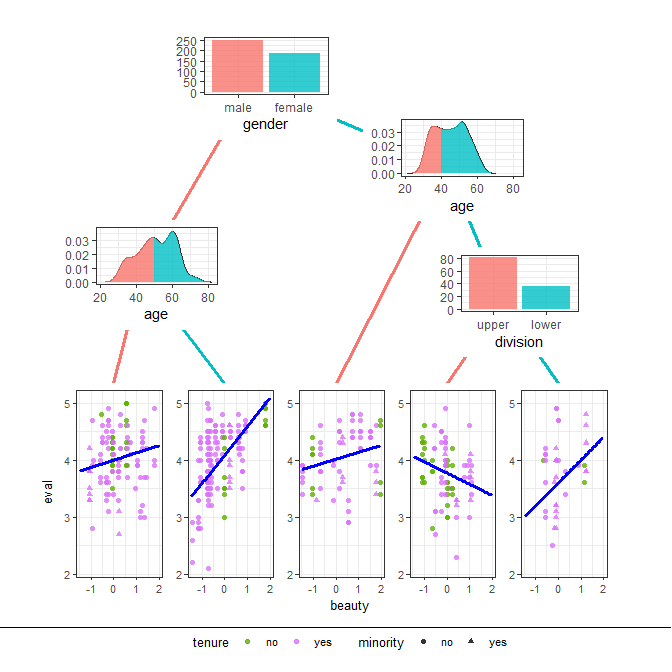# ggparty: Graphic Partying

#### 2019-07-15

ggparty aims to extend ggplot2 functionality to the partykit package. It provides the necessary tools to create clearly structured and highly customizable visualizations for tree-objects of the class 'party'.

# ggparty

Loading the ggparty package will also load partykit and ggplot2 and thereby provide all necessary functions.

library(ggparty)
#> Loading required package: ggplot2
#> Loading required package: partykit
#> Loading required package: grid
#> Loading required package: libcoin
#> Loading required package: mvtnorm

## Motivating Example

The following plot can be created fairly easily with ggparty. All it takes is an object of class party, some basic knowledge of ggplot2 and comprehension of the topics covered in this vignette.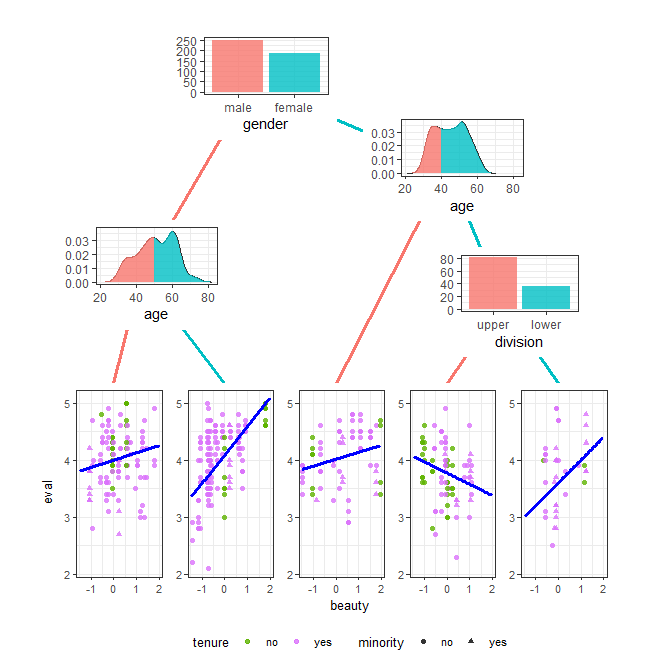The code used to create this plot can be found at the end of this document. But first things first.
Let’s recreate a simple example already used in the partykit vignette. If you are not familiar with the partykit you should definitely check it out before you work with this package.

data("WeatherPlay", package = "partykit")
sp_o <- partysplit(1L, index = 1:3)
sp_h <- partysplit(3L, breaks = 75)
sp_w <- partysplit(4L, index = 1:2)
pn <- partynode(1L, split = sp_o, kids = list(
partynode(2L, split = sp_h, kids = list(
partynode(3L, info = "yes"),
partynode(4L, info = "no"))),
partynode(5L, info = "yes"),
partynode(6L, split = sp_w, kids = list(
partynode(7L, info = "yes"),
partynode(8L, info = "no")))))
py <- party(pn, WeatherPlay)

## ggparty()

The ggparty() function takes a tree of class party and allows us to plot it with the help of the ggplot2 package. To make this possible, the 'party' object first needs to be transformed into a 'data.frame' and be passed to a ggplot() call. This is exactly what happens when we run ggparty().

is.ggplot(ggparty(py))

 TRUE


pander::pandoc.table(ggparty(py)$data[,1:16]) Table continues below id x y parent birth_order breaks_label info info_list 1 0.5 1 NA 0 NA NA NA 2 0.2 0.75 1 1 sunny NA NA 3 0.1 0.5 2 1 NA <= NA* 75 yes NA 4 0.3 0.5 2 2 NA > NA* 75 no NA 5 0.5 0.5 1 2 overcast yes NA 6 0.8 0.75 1 3 rainy NA NA 7 0.7 0.5 6 1 false yes NA 8 0.9 0.5 6 2 true no NA splitvar level kids nodesize p.value horizontal x_parent y_parent outlook 0 3 14 NA FALSE NA NA humidity 1 2 5 NA FALSE 0.5 1 NA 2 0 2 NA FALSE 0.2 0.75 NA 2 0 3 NA FALSE 0.2 0.75 NA 2 0 4 NA FALSE 0.5 1 windy 1 2 5 NA FALSE 0.5 1 NA 2 0 3 NA FALSE 0.8 0.75 NA 2 0 2 NA FALSE 0.8 0.75 ## Plot Data The first 16 columns of the 'data.frame' passed by ggparty() to ggplot() contain these values: • id… ID of the node • x… X coordinate of the node • y… Y coordinate of the node • parent… ID of node’s parent • birth_order… Position relative to parent. Goes from left to right. • breaks_label… String containing the corresponding split break of the parent’s split variable. • info… String containing the info of the node • info_list… List containing the info of the node if it was a list • splitvar… String containing the name of the Variable to split with. (only inner nodes) • level… At which level to draw the node. (0 = root) • kids… Number of node’s kids • nodesize… Number of rows in node’s data. • p.value… P value of model if present • horizontal… Logical - specifies whether the tree is to be drawn horizontally or vertically. Identical for all nodes. • x_parent… X coordinate of the node’s parent • y_parent… Y coordinate of the node’s parent The remaining columns contain lists of the node’s data and we will need geom_node_plot() to work with them. # Plotting a Tree Every **ggparty plot starts with a call to the eponymous ggparty() function which requires an object of class 'party'. To draw a tree we will need to add several of these components: ## Basic Building Blocks • geom_edge() draws the edges between the nodes • geom_edge_label() labels the edges with the corresponding split breaks • geom_node_label() labels the nodes with the split variable, node info or anything else. The shorthand versions of this geom geom_node_splitvar() and geom_node_info() have the correct defaults to write the split variables in the inner nodes or the info in the terminal nodes. • geom_node_plot() creates a custom ggplot at the location of the node In most cases we will probably want to draw at least edges, edge labels and node labels, so we will have to call the respective functions. The default mappings of geom_edge() and and geom_edge_label() ensure that lines between the related nodes are drawn and the corresponding split breaks are plotted at their centers. Since the text we want to print on the nodes differs depending on the kind of node, we will call geom_node_label twice. Once for the inner nodes, to plot the split variables and once for the terminal nodes to plot the info elements of the tree, which in this case contain the play decision. ggparty(py) + geom_edge() + geom_edge_label() + geom_node_label(aes(label = splitvar), ids = "inner") + # identical to geom_node_splitvar() + geom_node_label(aes(label = info), ids = "terminal")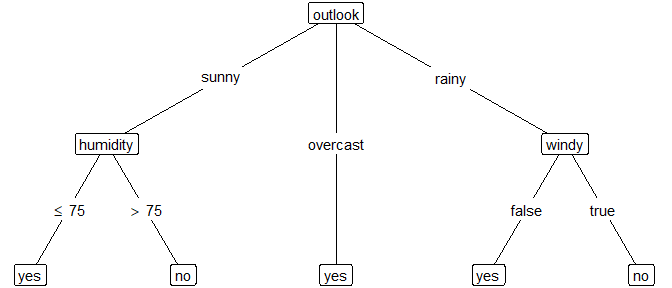# identical to geom_node_info()  Instead of adding geom_node_label() we can also add the convenience versions geom_node_splitvar() and geom_node_info() which contain the correct defaults to plot the split variables in the inner nodes and the info in the terminal nodes. Thanks to the ggplot2 mechanics we can now map different aspects of our plot to properties of the nodes. Whether that’s the best choice in this case is a different question. ggparty(py) + geom_edge() + geom_edge_label() + # map color to level and size to nodesize for all nodes geom_node_splitvar(aes(col = factor(level), size = nodesize)) + geom_node_info(aes(col = factor(level), size = nodesize))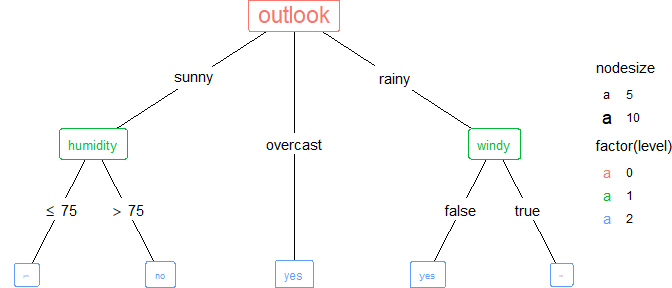We can create a horizontal tree simply by setting horizontal in ggparty() to TRUE. ggparty(py, horizontal = TRUE) + geom_edge() + geom_edge_label() + geom_node_splitvar() + geom_node_info()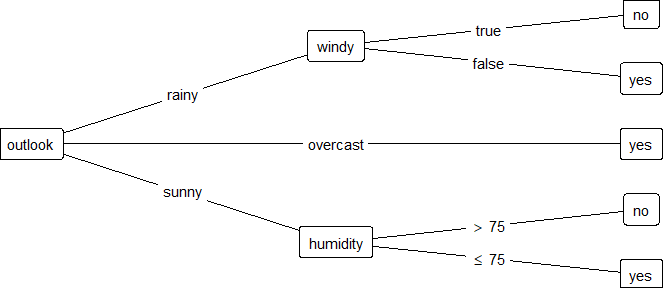## Additional Data This section is about extracting additional elements from the 'party' object or adding new data. If you just want to know how to make pretty plots, feel free to skip forward to the next section. If the default amount of elements extracted from the 'party' object is not enough for our purposes, there is a way to add more. Setting the argument add_vars of the ggparty() call we can specify what to extract and how to store it (affecting how we can use it later on). Let’s say we want to add for each node the information whether the split break is closed on the right. We can do this the following way: gg <- ggparty(py, add_vars = list(right = "$node$split$right"))
gg$data$right
#>  TRUE TRUE   NA   NA   NA TRUE   NA   NA

As we can see we need to pass a named 'list' to add_vars. The names of the elements of the list will become the names of the columns in the plot data and the elements of the list need to be either a 'character' string specifying how to extract the desired element from each node (as seen above) or a function that will be applied consecutively to each node and each row of the plot data. If we want to simply add something to the plot data, so that it can be accessed by base level geoms (geoms making up the tree) it has to be of length one like in the example above. The same result can of course be achieved using a 'function:'

gg <- ggparty(py, add_vars = list(right =
function(data, node) {
node$node$split$right } ) ) gg$data$right #>  TRUE TRUE NA NA NA TRUE NA NA But what if we want to add data to our node’s data so that it is simultaneously accessible through a single geom? One way to do it, is to name the list element with the prefix "nodedata_" and assign a 'function' which returns a 'list' for each node. It is important that the lists be of the same length as the lists created from the node’s data. I.e. the new data has to have the same number of observations as the node’s data since it needs to fit into one 'data.frame'. We are effectively adding columns to the node’s data. As we can see below, the plot data’s nodesize can be useful to make sure of this. Once we call geom_node_plot() this data will be readily available through gglist under its name (which we set for it as the name of the list element) without the prefix - just like all the node’s data. gg <- ggparty(py, add_vars = list(nodedata_x_dens = function(data, node) { list(density(node$data$temperature, n = data$nodesize)$x) } ) ) gg$data$nodedata_x_dens #> [] #>  53.53320 56.75887 59.98453 63.21019 66.43585 69.66151 72.88717 #>  76.11283 79.33849 82.56415 85.78981 89.01547 92.24113 95.46680 #> #> [] #>  57.31698 67.15849 77.00000 86.84151 96.68302 #> #> [] #>  63.73772 80.26228 #> #> [] #>  61.48648 78.50000 95.51352 #> #> [] #>  46.43918 64.47973 82.52027 100.56082 #> #> [] #>  60.61887 65.30943 70.00000 74.69057 79.38113 #> #> [] #>  62.33887 71.50000 80.66113 #> #> [] #>  59.73772 76.26228 The obvious limitation of this method is that the number of observations has to be identical to the nodesize. In this case we achieved this by setting n of density() to the nodesize. If we want to plot custom data of different dimensions we can simply supply it via the data argument of the geoms in gglist. Though in that case we won’t be able to access it simultaneously with the node’s data in the same geom. To ensure correct behaviour this 'data.frame' has to contain a column named id specifying the id of the node it belongs to. # Node Plots If we want to plot the data contained within the individual nodes of the tree, we need to add geom_node_plot() to our ggparty() call. To understand why this is necessary let’s reiterate what ggparty() does and how it uses the ggplot() function. Every ggplot() call needs a 'data.frame', so as we’ve seen above ggparty() creates one from the 'party' object. In this 'data.frame' every row corresponds to a node of the tree. Each column of this node’s data is stored as a 'list' in its own column. This way it is not usable by ggplot(), since ggplot() can’t handle lists inside its data. This is where geom_node_plot() comes into play and each instance of geom_node_plot() creates a completely separate ggplot() call after transforming all the columns containing lists of data (created by ggparty()) into a new 'data.frame' for the new separate ggplot() call. All the other columns of ggparty’s 'data.frame' (like kids, parent, etc.) get lost in this process, since usually we will not be interested in these when plotting the node data and they could potentially cause naming conflicts. In case we do want to use them, there is a fairly easy way to do so. So by default we can access anything that can be found in the data slot of the party object, the fitted_nodes and additionally if the 'party' object contains any, the fitted.values and the residuals of the included model. Now let’s take a look at a constparty object created from the same data. n1 <- partynode(id = 1L, split = sp_o, kids = lapply(2L:4L, partynode)) t2 <- party(n1, data = WeatherPlay, fitted = data.frame( "(fitted)" = fitted_node(n1, data = WeatherPlay), "(response)" = WeatherPlay$play,
check.names = FALSE),
terms = terms(play ~ ., data = WeatherPlay)
)
t2 <- as.constparty(t2)

To visualize the distribution of the variable play we will use the geom_node_plot() function. It allows us to show the data of each node in its separate plot. For this to work, we have to specify the argument gglist. Basically we have to provide a 'list' of all the 'gg' components we would add to a ggplot() call on the data element of a node.

ggplot(t2$data) + geom_bar(aes(x = "", fill = play), position = position_fill()) + xlab("play")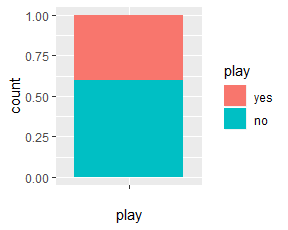So if we were to use the above code to create the desired plot for one node, we can instead pass a 'list' of the two components to gglist and geom_node_plot will create a version of it for every specified node (per default the terminal nodes). Keep in mind, that since it’s a 'list' we need to use "," instead of "+" to combine the components. ggparty(t2) + geom_edge() + geom_edge_label() + geom_node_splitvar() + # pass list to gglist containing all ggplot components we want to plot for each # (default: terminal) node geom_node_plot(gglist = list(geom_bar(aes(x = "", fill = play), position = position_fill()), xlab("play")))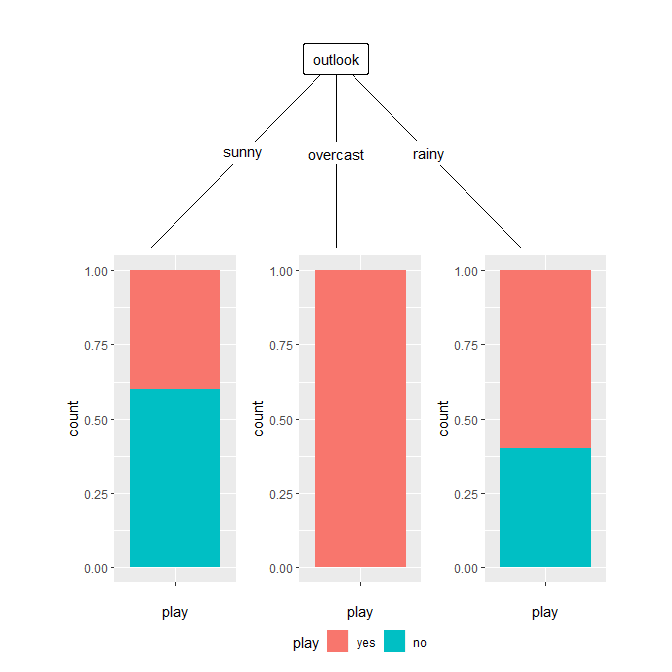## Axes and Legends Setting shared_axis_labels to TRUE allows us to use the space more efficiently and legend_separator = TRUE draws a line between the tree and the legend. ggparty(t2) + geom_edge() + geom_edge_label() + geom_node_splitvar() + geom_node_plot(gglist = list(geom_bar(aes(x = "", fill = play), position = position_fill()), xlab("play")), # draw only one label for each axis shared_axis_labels = TRUE, # draw line between tree and legend legend_separator = TRUE )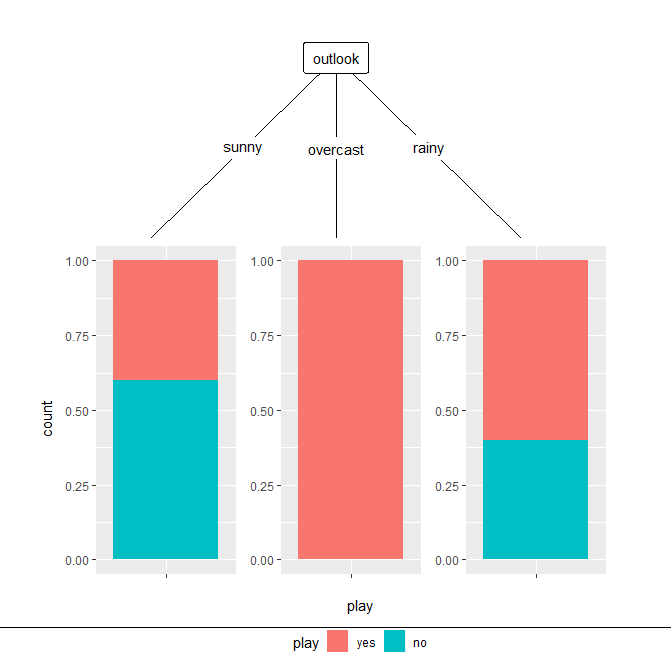Setting shared_legend to FALSE draws an individual legend at each plot instead of one common one at the bottom of the plot. This might be necessary if we use multiple different geom_node_plots() which lead to various legends. In case we want to remove the legend all together (i.e. theme(legend.position = "none")) shared_legend has to be set to FALSE. ggparty(t2) + geom_edge() + geom_edge_label() + geom_node_splitvar() + geom_node_plot(gglist = list(geom_bar(aes(x = "", fill = play), position = position_fill()), xlab("play")), # draw individual legend for each plot shared_legend = FALSE )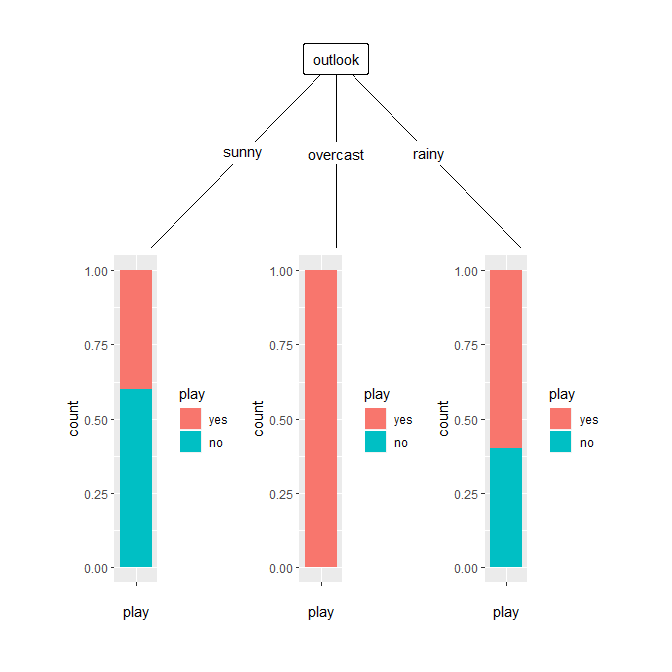Thanks to the versatility of ggplot2 we are also very flexible in creating these node plots. For example the barplot can be easily changed into a pie chart. The argument size of geom_node_plot() can be set to "nodesize" which changes the size of the node plot relative to the number of observations in the respective node. ggparty(t2) + geom_edge() + geom_edge_label() + geom_node_splitvar() + # draw pie charts with their size relative to nodesize geom_node_plot(gglist = list(geom_bar(aes(x = "", fill = play), position = position_fill()), coord_polar("y"), theme_void()), size = "nodesize")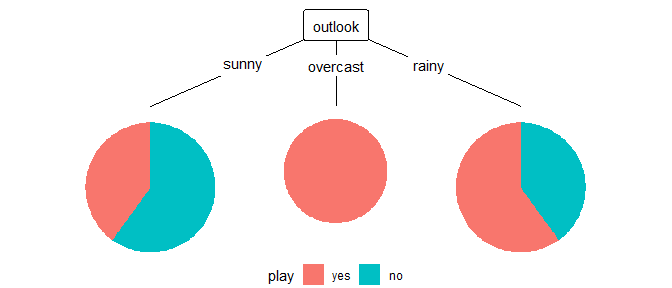## Predictions If the party object contains a model with only one predictor we can use the argument predict to choose to show a prediction line. Additional arguments for the geom_line() drawing this line can be passed via predict_gpar. So let’s take a look at this 'lmtree' containing linear models explaining eval with beauty. data("TeachingRatings", package = "AER") tr <- subset(TeachingRatings, credits == "more") tr_tree <- lmtree(eval ~ beauty | minority + age + gender + division + native + tenure, data = tr, weights = students, caseweights = FALSE) ggparty(tr_tree) + geom_edge() + geom_edge_label() + geom_node_splitvar() + geom_node_plot(gglist = list(geom_point(aes(x = beauty, y = eval, col = tenure, shape = minority), alpha = 0.8), theme_bw(base_size = 10)), shared_axis_labels = TRUE, legend_separator = TRUE, # predict based on variable predict = "beauty", # graphical parameters for geom_line of predictions predict_gpar = list(col = "blue", size = 1.2) )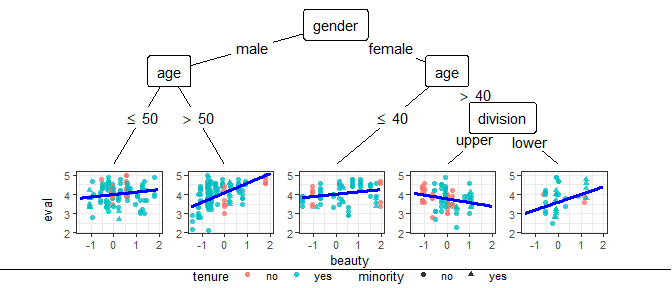In case we want to generate predictions for a more complicated model, we need to do this beforehand and pass the new data through the data argument inside geom_node_plot()’s gglist. First the tree of class 'party' is created using the partykit infrastructure. data("GBSG2", package = "TH.data") GBSG2$time <- GBSG2$time/365 library("survival") wbreg <- function(y, x, start = NULL, weights = NULL, offset = NULL, ...) { survreg(y ~ 0 + x, weights = weights, dist = "weibull", ...) } logLik.survreg <- function(object, ...) structure(object$loglik, df = sum(object$df), class = "logLik") gbsg2_tree <- mob(Surv(time, cens) ~ horTh + pnodes | age + tsize + tgrade + progrec + estrec + menostat, data = GBSG2, fit = wbreg, control = mob_control(minsize = 80)) So in this case we want to create a sequence over the range of the metric variable pnodes and combine it once with the first level of the binary variable horTh and once with the second. Using this data we then (in this case) need to generate predictions of the type "quantile" with p set to 0.5. The function get_predictions() can help us with the second part since it applies a newdata function defined by us to each node and returns a suitable 'data.frame'. If we want to use it, we need to supply the 'party' object, a function that creates the new data from each node’s data and optionally predict_arg, additional arguments to pass to the predict() call. # function to generate newdata for predictions generate_newdata <- function(data) { z <- data.frame(horTh = factor(rep(c("yes", "no"), each = length(data$pnodes))),
pnodes = rep(seq(from = min(data$pnodes), to = max(data$pnodes),
length.out = length(data$pnodes)), 2)) z$x <- model.matrix(~ ., data = z)
z}

# convenience function to create dataframe for predictions
pred_df <- get_predictions(gbsg2_tree,
# IMPORTANT to set same ids as in geom_node_plot
# later used for plotting
ids = "terminal",
newdata_fun = generate_newdata,
predict_arg = list(type = "quantile",
p = 0.5)
)

The 'data.frame' created this way can then be passed to any 'gg' component in geom_node_plot()’s gglist. In this case we want to draw a line for both values of horTh and separate them by color.

ggparty(gbsg2_tree, terminal_space = 0.8, horizontal = TRUE) +
geom_edge() +
geom_node_splitvar() +
geom_edge_label() +
geom_node_plot(
gglist = list(geom_point(aes(y = Surv(time, cens).time,
x = pnodes,
col = horTh),
alpha = 0.6),
# supply pred_df as data argument of geom_line
geom_line(data = pred_df,
aes(x = pnodes,
y = prediction,
col = horTh),
size = 1.2),
theme_bw(),
ylab("Survival Time")
),
ids = "terminal", # not necessary since default
shared_axis_labels = TRUE
)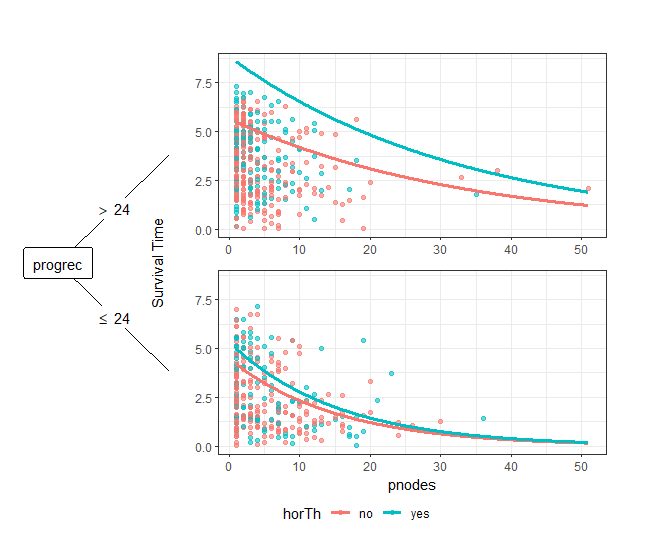## Potential Pitfalls

### Combining 'gg' Components in gglist with "+"

The object passed to gglist has to be a 'list' and therefore we must not use "+" to combine the components of a geom_node_plot() but instead ",".

### Passing Components at the Wrong Place

As we now know, each geom_node_plot() is basically a completely separate plot with its own arguments and specifications which are independent from the base plot of the tree (i.e. the ggparty call with edges, labels, etc.). For that reason, if for example, we want to remove the legend of a geom_node_plot() we must not pass it at the base level (as a component of the tree) but inside the gglist of the geom_node_plot().

# Node Labels

geom_node_label() is a modified version of ggplot2’s geom_label() which allows for multi-line labels. However the basic functionality of geom_label() is still present. This means that if we are content with uniform aesthetics for the whole label, we can simply use geom_node_label() as we would geom_label() with the only difference, that x and y are already mapped per default to the nodes coordinates.

If we want to have to specify even less mappings, we can use geom_node_splitvar() and geom_node_info(). These are wrappers of geom_node_label() with the respective defaults to plot the splitvar in the inner nodes or the info in the terminal nodes.

## Multi-Line Labels

geom_node_label() allows us to create multiline labels and specify individual graphical parameters for each line. To do this, we must not map anything to label in the aes() passed to mapping, but instead pass a 'list' of aes() to the argument line_list. The order of the 'list' is the same as the order in which the lines will be printed. Additionally we have to pass a 'list' to line_gpar. This list must be the same length as line_list and contain separately named 'lists' of graphical parameters. If we don’t want to change anything for a specific line, the respective ’list' has to be an empty 'list'.

Mapping with the mapping argument of geom_node_label() still works and affects all lines and the border together. The line specific graphical arguments in line_gpar can be used to overwrite these mappings.
Additionally to the usual aesthetic parameters we would use for ggplot’s geom_label() we can pass parse and alignment through line_gpar. Parse is equivalent to the behaviour of geom_label() and alignment enables us to position the text at the left or right label border.

All other mappings in line_list will be ignored. It is not possible to map other line specific aesthetics to variables. It is only possible to map the aesthetics of the complete label to variables and overwrite specific lines with fixed values in line_gpar. (In essence replicating the condition of mapping only one line to a variable, but we won’t be able to do this for multiple lines with different mappings).

This may seem very convoluted, but keep in mind, that we only have to go through this process if we want to address the graphical parameters of specific lines.

### Example

To create a tree consisting of inner nodes labeled by their split variable and terminal nodes labeled by their coefficients we can use the code found below.

First we need to extract the coefficients with the help of the add_vars argument of ggparty(). This step is necessary so that we can later access them by the names given to them in the 'list' supplied to add_vars.

Since we want to plot different elements in the inner and terminal nodes, we need to add geom_node_label() twice. The first call is for the inner nodes. With the aes() passed to mapping we map the color of the labels to the splitvar of the node.

For this tree we want to display the split variable in the first line, then the p-value in scientific notation in the second line, the third line is just a spacer therefore empty and the fourth and last line is supposed to show the ID of the node. We specify the aesthetics we want to override in line_gpar. Using the third line as a spacer and setting alignment to “left” we can position the id of the node at the bottom left corner of the labels.
Correspondingly we can plot the labels for the terminal nodes.

ggparty(tr_tree,
terminal_space = 0,
add_vars = list(intercept = "$node$info$coefficients", beta = "$node$info$coefficients")) +
geom_edge(size = 1.5) +
geom_edge_label(colour = "grey", size = 4) +
# first label inner nodes
geom_node_label(# map color of complete label to splitvar
mapping = aes(col = splitvar),
# map content to label for each line
line_list = list(aes(label = splitvar),
aes(label = paste("p =",
formatC(p.value,
format = "e",
digits = 2))),
aes(label = ""),
aes(label = id)
),
# set graphical parameters for each line in same order
line_gpar = list(list(size = 12),
list(size = 8),
list(size = 6),
list(size = 7,
col = "black",
fontface = "bold",
alignment = "left")
),
# only inner nodes
ids = "inner") +
# next label terminal nodes
geom_node_label(# map content to label for each line
line_list = list(
aes(label = paste("beta == ", round(intercept, 2))),
aes(label = paste("beta == ",round(beta, 2))),
aes(label = ""),
aes(label = id)
),
# set graphical parameters for each line in same order
line_gpar = list(list(size = 12, parse = T),
list(size = 12, parse = T),
list(size = 6),
list(size = 7,
col = "black",
fontface = "bold",
alignment = "left")),
ids = "terminal",
# nudge labels towards bottom so that edge labels have enough space
# alternatively use shift argument of edge_label
nudge_y = -.05) +
# don't show legend for splitvar mapping to color since self-explanatory
theme(legend.position = "none") +
# html_documents seem to cut off a bit too much at the edges so set limits manually
coord_cartesian(xlim = c(0, 1), ylim = c(-0.1, 1.1))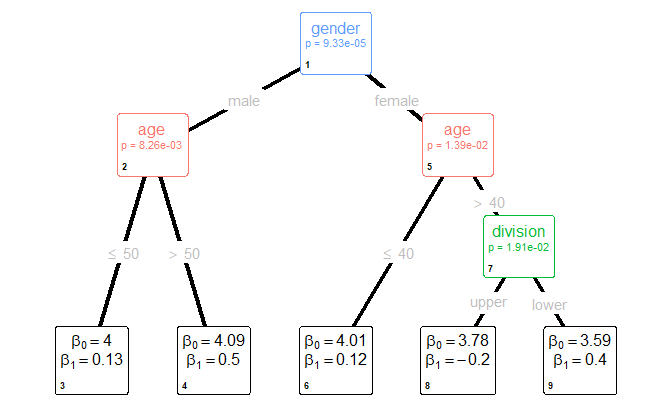# Layout

## Nodes

## Boston housing data
data("BostonHousing", package = "mlbench")
BostonHousing <- transform(BostonHousing,
chas = factor(chas, levels = 0:1, labels = c("no", "yes")),
rad = factor(rad, ordered = TRUE))

## linear model tree
bh_tree <- lmtree(medv ~ log(lstat) + I(rm^2) | zn +
indus + chas + nox + age + dis + rad + tax + crim + b + ptratio,
data = BostonHousing, minsize = 40)

Let’s take a look at ggparty()’s layout system with the help of this 'lmtree' based on BostonHousing data set from mlbench.

# terminal space specifies at which value of y the terminal plots begin
bh_plot <- ggparty(bh_tree, terminal_space = 0.5) +
geom_edge() +
geom_edge_label() +
geom_node_splitvar() +
# plot first row
geom_node_plot(gglist = list(
geom_point(aes(y = medv, x = log(lstat), col = chas),
alpha = 0.6)),
# halving the height shrinks plots towards the top
height = 0.5) +
# plot second row
geom_node_plot(gglist = list(
geom_point(aes(y = medv, x = I(rm^2), col = chas),
alpha = 0.6)),
height = 0.5,
# move -0.25 y to use the bottom half of the terminal space
nudge_y = -0.25)

bh_plot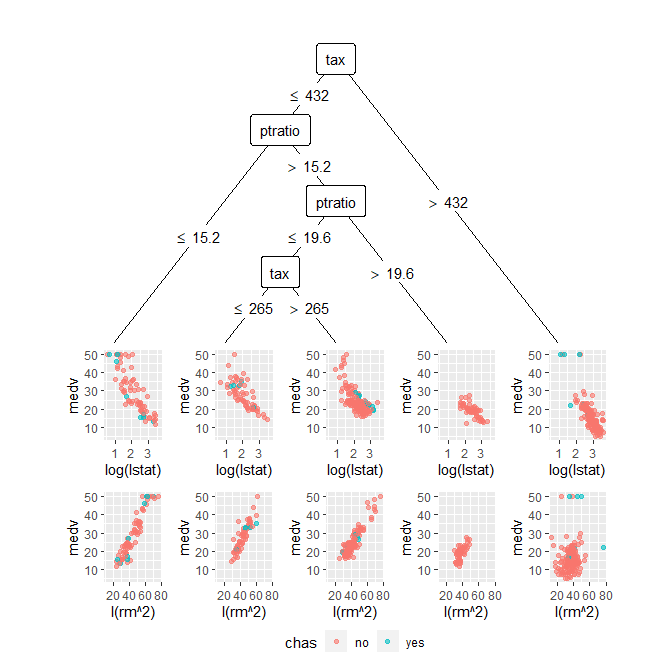ggparty() positions all the nodes within the unit square. For vertical trees the root is always at (0.5, 1), for horizontal ones it is at (0, 0.5). The argument terminal_size specifies how much room should be left for terminal plots. The default value depends on the depth of the supplied tree. The terminal nodes are placed at this value and in case labels are drawn, they are drawn there. In case plots are to be drawn their top borders are aligned to this value, i.e. the terminal plots just is not "center" but "top". Therefore reducing the height of a terminal node shrinks it towards the top.

So if we want to plot multiple plots per node we have to keep this in mind and can achieve this for example like this.
The first geom_node_plot() only takes the argument height = 0.5 which halves its size and effectively makes it occupy only the upper half of the area it would normally do. For the second geom_node_plot() we also specify the size to be 0.5 but additionally we have to specify nudge_y. Since the terminal space is set to be 0.5, we know that the first plot now spans from 0.5 to 0.25. So we want to move the line where to place the second plot to 0.25, i.e. nudge it from 0.5 by -0.25.

Changing the theme from the default theme_void to one for which gridlines are drawn allows us to see the layout structure described above.

bh_plot + theme_bw()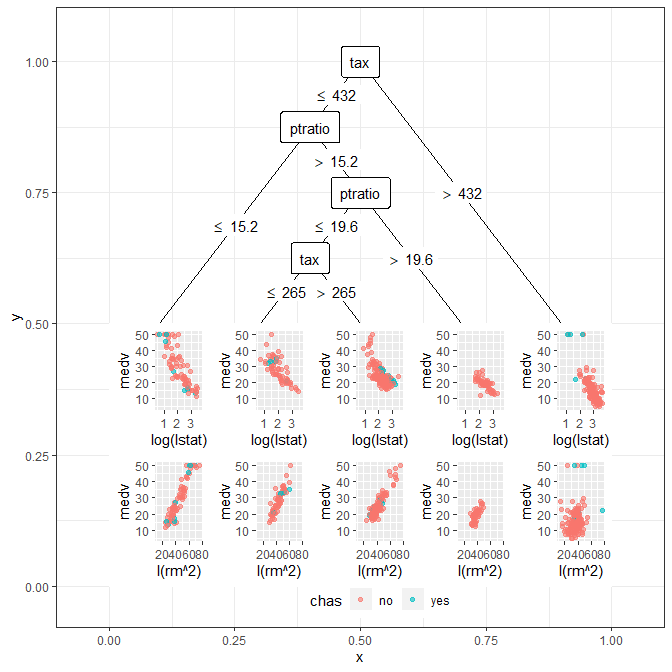We can use this information to manually set the positions of nodes. To do this we must pass a 'data.frame' containing the columns id, x and y to the layout argument of ggparty().

ggparty(bh_tree, terminal_space = 0.5,
# id specifies node; x and y values need to be between 0 and 1
layout = data.frame(id = c(1, 2),
x = c(0.7, 0.3),
y = c(1, 0.9))
) +
geom_edge() +
geom_edge_label() +
geom_node_splitvar() +
geom_node_plot(gglist = list(
geom_point(aes(y = medv, x = log(lstat), col = chas),
alpha = 0.6)),
height = 0.5) +
geom_node_plot(gglist = list(
geom_point(aes(y = medv, x = I(rm^2), col = chas),
alpha = 0.6)),
height = 0.5,
nudge_y = -0.25) +
theme_bw()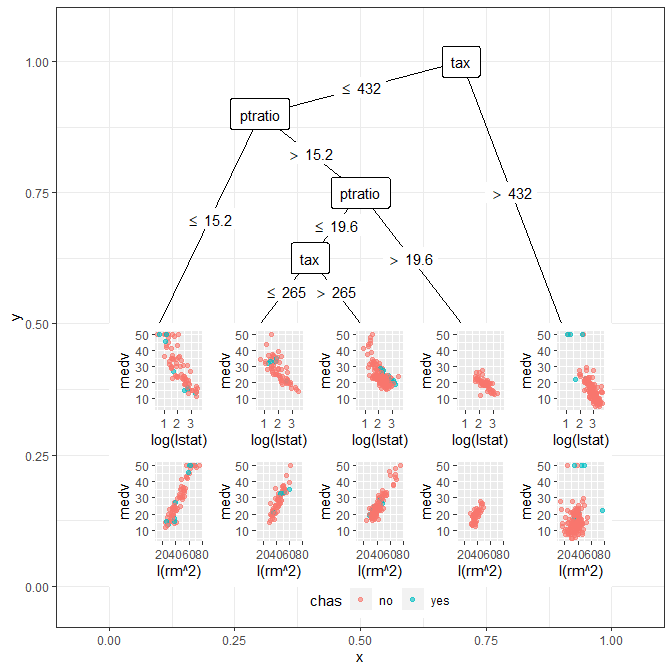## Axes, Legends and Limits

As mentioned the nodes of the tree should always be positioned inside the unit square. In case of a shared legend and no shared axis labels, it is plotted at (0.5, -0.05) with just = "top". In case shared axis labels are used, just changes to "bottom" (i.e. the legend shifts approximately 0.05 units downwards), and the x axis label takes its position. Furthermore the shared y axis label will be plotted outside the unit square. I.e. it can often be the case that limits based on the unit square will not be sufficient to capture all elements and ggparty() should be able to automatically cope with these situations.
In case you should need to adjust the x and y limits anyway, be advised to use coord_cartesian(xlim, ylim) instead of ylim and xlim since the latter can easily lead to unintended consequences by removing observations outside the plot limits.

# Autoplot Methods

The objects used in this document can also be plotted using the autoplot methods provided by ggparty.

autoplot(py)autoplot(t2)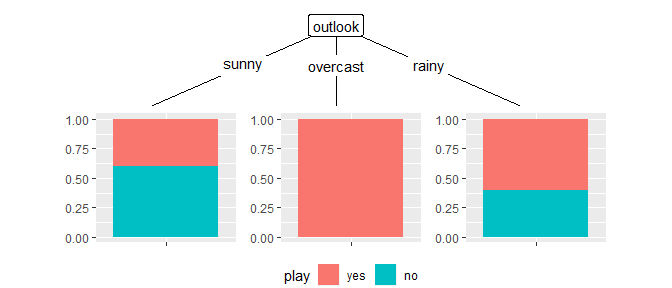autoplot(bh_tree, plot_var = "log(lstat)", show_fit = FALSE)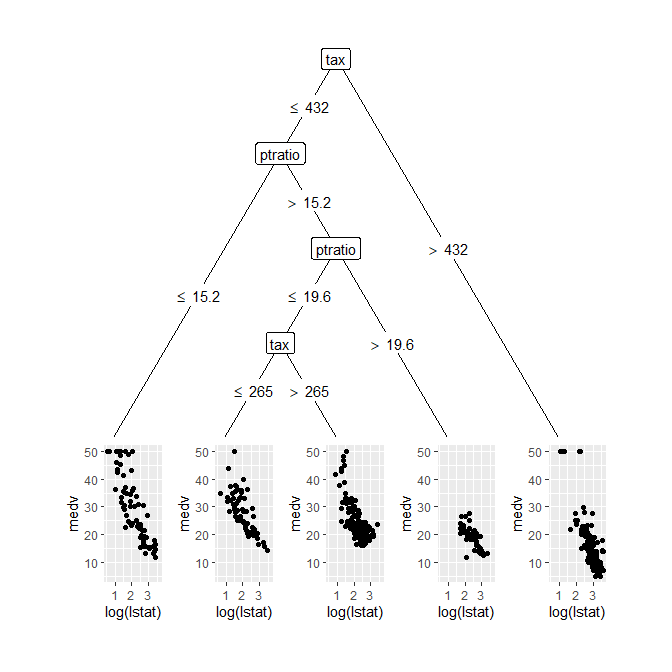autoplot(bh_tree, plot_var = "I(rm^2)", show_fit = TRUE)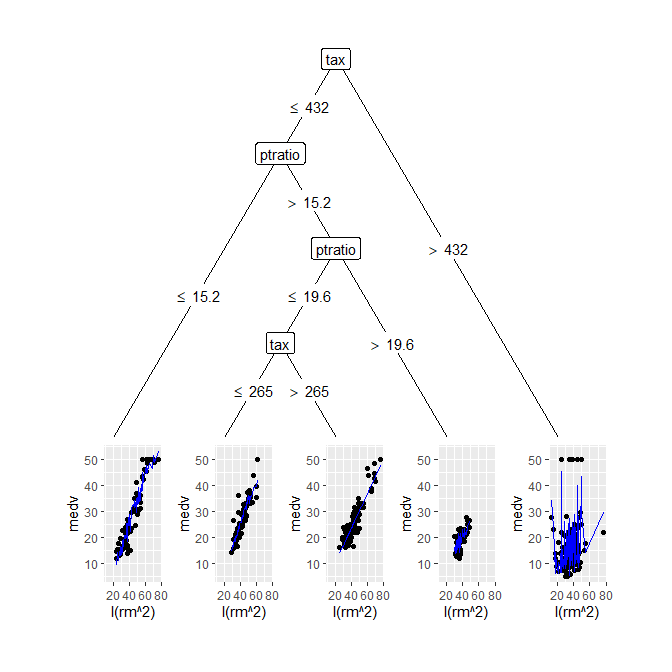autoplot(gbsg2_tree, plot_var = "pnodes")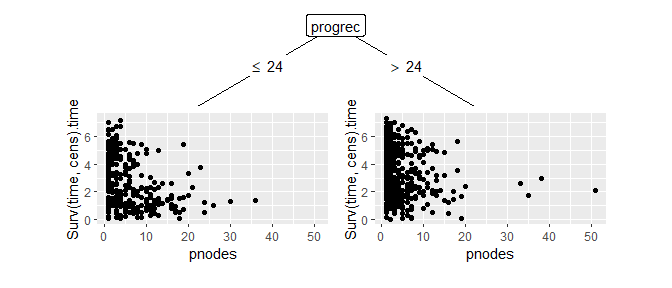autoplot(tr_tree)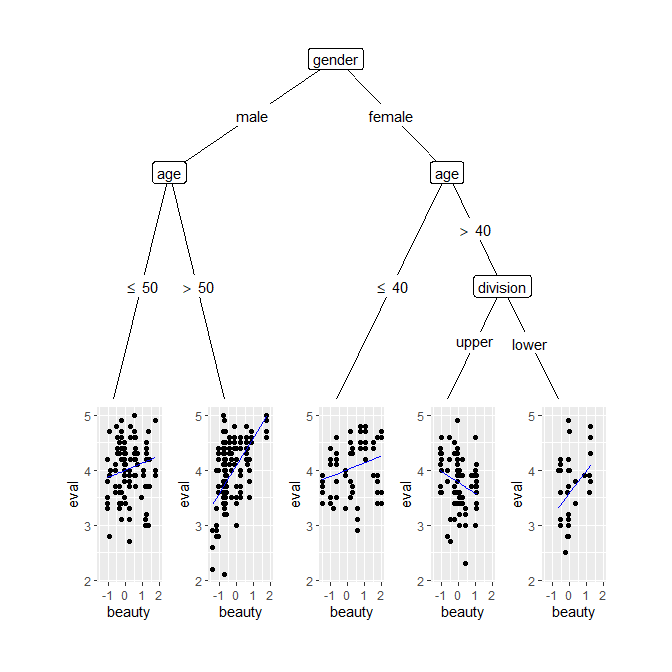# Examples

Using the techniques covered in this document we should now be able to plot quite nice trees of any 'party' object without much effort. Let’s take a look at a few possibilities using the tr_tree we are already familiar with.


asterisk_sign <- function(p_value) {
if (p_value < 0.001) return(c("***"))
if (p_value < 0.01) return(c("**"))
if (p_value < 0.05) return(c("*"))
else return("")
}

ggparty(tr_tree,
terminal_space = 0.5) +
geom_edge(size = 1.5) +
geom_edge_label(colour = "grey", size = 4) +
# plot fitted values against residuals for each terminal model
geom_node_plot(gglist = list(geom_point(aes(x = fitted_values,
y = residuals,
col = tenure,
shape = minority),
alpha = 0.8),
geom_hline(yintercept = 0),
theme_bw(base_size = 10)),
# y scale is fixed for better comparability,
# x scale is free for effecient use of space
scales = "free_x",
ids = "terminal",
shared_axis_labels = TRUE
) +
# label inner nodes
geom_node_label(aes(col = splitvar),
# label nodes with ID, split variable and p value
line_list = list(aes(label = paste("Node", id)),
aes(label = splitvar),
aes(label = asterisk_sign(p.value))
),
# set graphical parameters for each line
line_gpar = list(list(size = 8, col = "black", fontface = "bold"),
list(size = 12),
list(size = 8)
),
ids = "inner") +
# add labels for terminal nodes
geom_node_label(aes(label = paste0("Node ", id, ", N = ", nodesize)),
fontface = "bold",
ids = "terminal",
size = 3,
# 0.01 nudge_y is enough to be above the node plot since a terminal
# nodeplot's top (not center) is at the node's coordinates.
nudge_y = 0.01) +
theme(legend.position = "none")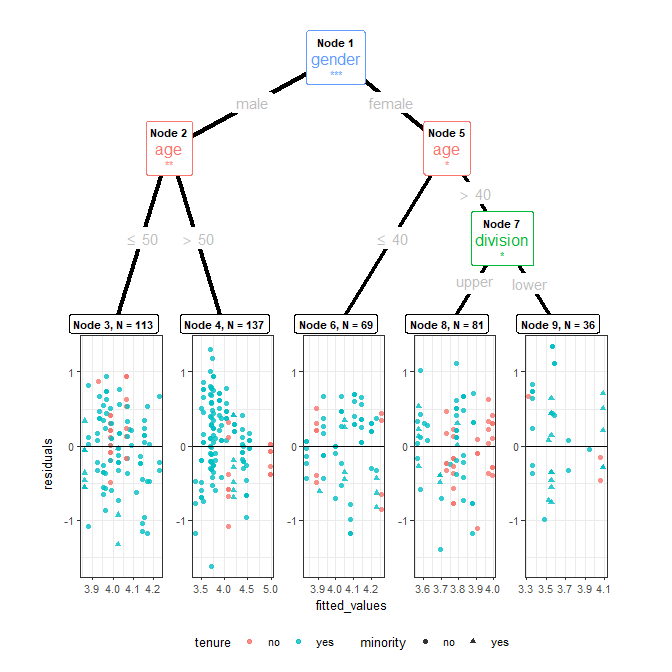This is the code for the example at the beginning of the document.

# create dataframe with ids, densities and breaks
# since we are going to supply the data.frame directly to a geom inside gglist,
# we don't need to worry about the number of observations per id and only data for the ids
# used by the respective geom_node_plot() needs to be generated (2 and 5 in this case)
dens_df <- data.frame(x_dens = numeric(), y_dens = numeric(), id = numeric(), breaks = character())
for (id in c(2, 5)) {
x_dens <- density(tr_tree[id]$data$age)$x y_dens <- density(tr_tree[id]$data$age)$y
breaks <- rep("left", length(x_dens))
if (id == 2) breaks[x_dens > 50] <- "right"
if (id == 5) breaks[x_dens > 40] <- "right"
dens_df <- rbind(dens_df, data.frame(x_dens, y_dens, id, breaks))
}

# adjust layout so that each node plot has enough space
ggparty(tr_tree, terminal_space = 0.4,
layout = data.frame(id = c(1, 2, 5, 7),
x = c(0.35, 0.15, 0.7, 0.8),
y = c(0.95, 0.6, 0.8, 0.55))) +
# map color of edges to birth_order (order from left to right)
geom_edge(aes(col = factor(birth_order)),
size = 1.2,
alpha = 1,
# exclude root so it doesn't count as it's own colour
ids = -1) +
# density plots for age splits
geom_node_plot(ids = c(2, 5),
gglist = list( # supply dens_df and plot line
geom_line(data = dens_df,
aes(x = x_dens,
y = y_dens),
show.legend = FALSE,
alpha = 0.8),
# supply dens_df and plot ribbon, map color to breaks
geom_ribbon(data = dens_df,
aes(x = x_dens,
ymin = 0,
ymax = y_dens,
fill = breaks),
show.legend = FALSE,
alpha = 0.8),
xlab("age"),
theme_bw(),
theme(axis.title.y = element_blank())),
size = 1.5,
height = 0.5
) +
# plot bar plot of gender at root
geom_node_plot(ids = 1,
gglist = list(geom_bar(aes(x = gender, fill = gender),
show.legend = FALSE,
alpha = .8),
theme_bw(),
theme(axis.title.y = element_blank())),
size = 1.5,
height = 0.5
) +
# plot bar plot of division for node 7
geom_node_plot(ids = 7,
gglist = list(geom_bar(aes(x = division, fill = division),
show.legend = FALSE,
alpha = .8),
theme_bw(),
theme(axis.title.y = element_blank())),
size = 1.5,
height = 0.5
) +
# plot terminal nodes with predictions
geom_node_plot(gglist = list(geom_point(aes(x = beauty,
y = eval,
col = tenure,
shape = minority),
alpha = 0.8),
theme_bw(base_size = 10),
scale_color_discrete(h.start = 100)),
shared_axis_labels = TRUE,
legend_separator = TRUE,
predict = "beauty",
predict_gpar = list(col = "blue",
size = 1.1)) +
# remove all legends from top level since self explanatory
theme(legend.position = "none")